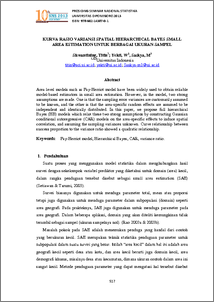# KURVA RASIO VARIANSI SPATIAL HIERARCHICAL BAYES SMALL AREA ESTIMATION UNTUK BERBAGAI UKURAN SAMPEL

UNSPECIFIED (2013) KURVA RASIO VARIANSI SPATIAL HIERARCHICAL BAYES SMALL AREA ESTIMATION UNTUK BERBAGAI UKURAN SAMPEL. PROSIDING SEMINAR NASIONAL STATISTIKA UNIVERSITAS DIPONEGORO 2013 . pp. 517-526. ISSN ISBN: 978-602-14387-0-1Preview
PDF
876Kb

## Abstract

Area level models such as Fay-Herriot model have been widely used to obtain reliable model-based estimators in small area estimation. However, in the model, two strong assumptions are made. One is that the sampling error variances are customarily assumed to be known, and the other is that the area-specific random effects are assumed to be independent and identically distributed. In this paper, we propose full hierarchical Bayes (HB) models which relax these two strong assumptions by constructing Gaussian conditional autoregressive (CAR) models on the area-specific effects to induce spatial correlation, and assuming the sampling variances unknown. Curve relationship between success proportion to the variance ratio showed a quadratic relationship. Keywords: Fay-Herriot model, Hierarchical Bayes, CAR, variance ratio.

Item Type: Article Q Science > Q Science (General) Faculty of Science and Mathematics > Department of Statistics 40351 INVALID USER 21 Oct 2013 16:27 21 Oct 2013 16:27

Repository Staff Only: item control page